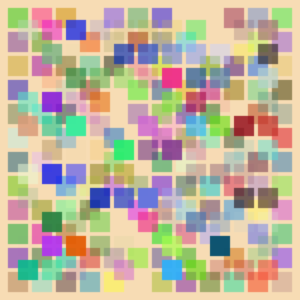# Tag Archives: math.stackexchange

## (But there are some positive changes that can be made)This is the final chapter in my series about the state of internet fora, and Math.SE and StackOverflow in particular. The previous chapters are Challenges Facing Community Cohesion and Ghosts of Forums Past. Unlike the previous entries, this also sits on Meta.Math.SE (and was posted there a week before here). (As I write this as a moderator of Math.SE, I refer to the Math.SE community as “we”, “us”, and “our” community).

A couple of weeks ago, there was a proposal on meta.Math.SE to introduce a third level of math site to the SE network. Many members of the the MathSE community have reacted very positively to this proposal, to the extent that even some of the moderators have considered throwing their weight behind it.

But a NoviceMathSE site would be doomed to fail, and such a separation would not solve the underlying problems facing the site.

To explain my point of view, we need to examine more closely the arguments in favor of NoviceMathSE.

Posted in Mathematics, SE | Tagged , | Leave a comment

## An elementary proof of when 2 is a quadratic residue

This has been a week of asking and answering questions from emails, as far as I can see. I want to respond to two questions publicly, though, as they’ve some interesting value. So this is the first of a pair of blog posts. One is a short and sweet elementary proof of when $2$ is a quadratic residue of a prime, responding to Moschops’s comments on an earlier blog post. But to continue my theme of some good and some bad, I’d also like to consider the latest “proof” of the Goldbach conjecture (which I’ll talk about in the next post tomorrow). More after the fold:

Posted in Expository, Math.NT, Mathematics, SE | | 7 Comments

## A MSE Collection: Topology Book References

Today, yet another question was posted on Math.Stackexchange (this time by the new user avatar) asking for topology references. This has been asked a few times before, but somehow the answers are a little bit different. So, as if I were responding to Ramana Venkata’s post on the meta about a consolidated topology resource, based upon the answers at MSE, and to facilitate topology references in the future, I am writing this blog post.

To be clear, this is a compilation of much (not all) of the discussion in the following questions (and their answers): best book for topology? by jgg, Can anybody recommend me a topology textbook? by henryforever14, choosing a topology text by A B, Introductory book on Topology by someguy, Reference for general-topology by newbie, Learning Homology and Cohomology by Refik Marul, What is a good Algebraic topology reference text? by babgen, Learning Roadmap for Algebraic Topology by msnaber, What algebraic topology book to read after Hatcher’s? by weylishere, Best Algebraic Topology book/Alternative to Allen Hatcher free book? by simplicity, and Good book on homology by yaa09d. And I insert my own thoughts and resources, when applicable. Ultimately, this is a post aimed at people beginning to learn topology, perhaps going towards homology and cohomology (rather than towards a manifolds-type, at least for now).

Posted in Math.AT, Mathematics, SE | | 1 Comment

## Reading Math

First, a recent gem from MathStackExchange:

Task: Calculate $\displaystyle \sum_{i = 1}^{69} \sqrt{ \left( 1 + \frac{1}{i^2} + \frac{1}{(i+1)^2} \right) }$ as quickly as you can with pencil and paper only.

Yes, this is just another cute problem that turns out to have a very pleasant solution. Here’s how this one goes. (If you’re interested – try it out. There’s really only a few ways to proceed at first – so give it a whirl and any idea that has any promise will probably be the only idea with promise).

Posted in Expository, Georgia Tech, Mathematics, SE, Story | | 10 Comments

## From the Exchange

I speak of Math Stackexchange frequently for two reasons: because it is fantastically interesting and because I waste inordinate amounts of time on it. But I would like to again share some of the more interesting things from the exchange here.

Posted in Mathematics, SE, Story | | Leave a comment